# How to Add a Comma after the First Word in Each Cell in Excel?

Adding commas manually in specific places within cells may be a pretty good idea when you deal with numerous cells inside your spreadsheet data. After the first word in a list that was randomly produced in Excel, it is highly typical for there to be an absence of commas in the data. This is particularly true in situations in which the data is copied from word editors. but, when you deal with a vast amount of data, you have to discover a better approach to do it or come up with a different way to do it manually.

You have the following methods to choose from if you want to insert a comma after the first word in each cell in Excel. Those are

• Using REPLACE function

• Using SUBSTITUTE function

Let’s see both the methods one by one.

## Using REPLACE Function

When you are familiar with the location and length of the characters whose appearance you wish to change, you should use the REPLACE function. This is the case when you want to change their appearance.

The formula to add comma after first word is as follows −

=REPLACE (the cell in which comma will be added, FIND(" ",the cell in which comma will be added),0,",")

Let’s see the following steps to use REPLACE function to insert a comma after the first word in a cell.

### Step 1

We have the following data in an Excel sheet. See the following image in which the comma will be added.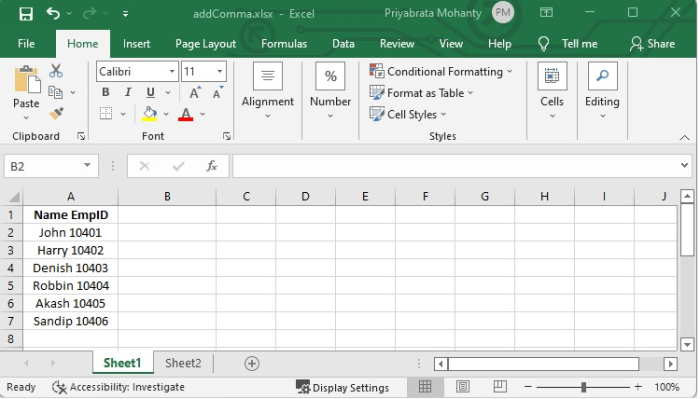In our spreadsheet, we have some employee names and employee IDs in one cell.

### Step 2

We want to separate the employee name and employeeId with comma using the below given formula.

=REPLACE(A1,FIND(" ",A1),0,",")

Refer the following image to understand how it's done.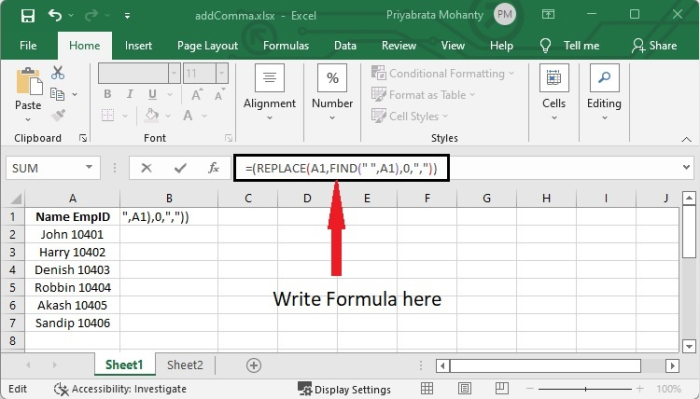In this example, first select one cell and the add the formula given above to separate comma. Entering the formula will produce the result as follows.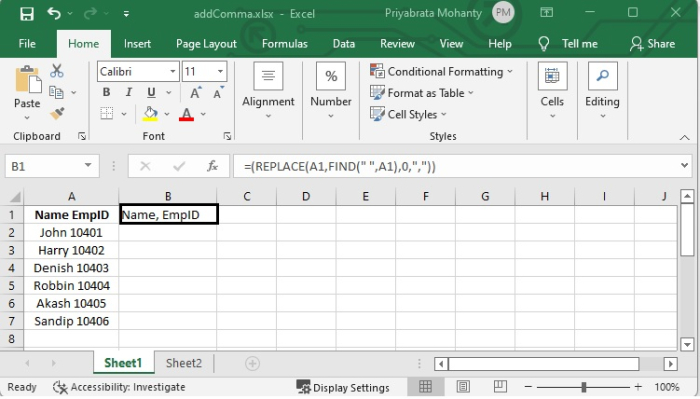Here, the FIND function will return the place in the text string "Name EmpID" that corresponds to the beginning of the space character. Given that the answer is 5, it can be concluded that the initial space in "Name" can be found in the fifth position. After that, Name, EmpID is the final result you should see after using the REPLACE function to move the comma to the fifth position in "Name, EmpId" as seen above.

### Step 3

To reflect this formula in other cells, select that B1 cell and drag it to the specified column below B1 cell and you will get the required result.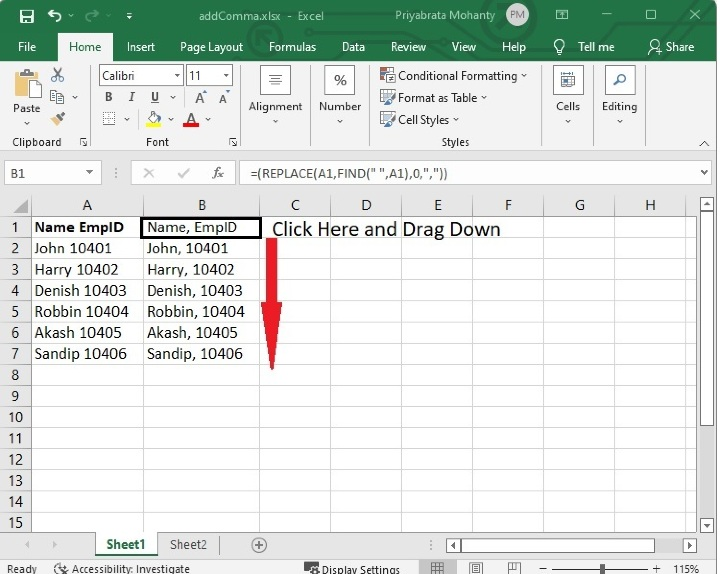## Using SUBSTITUTE Function

Excel's SUBSTITUTE function is a type of function which can be used to search for and replace certain text strings. If you want to replace an old text with a new one, this function will be quite helpful for you to employ. It replaces particular text in a string of text, means it inserts new content in place of the previous text in a string of text.

The formula to use SUBSTITUTE function is given below.

=SUBSTITUTE(cell, old text, new text, number of occurrences)

### Step 1

In our case to add comma after first word in cell, we will replace the first occurrence of space with comma (,). See the below given formula.

=SUBSTITUTE(A1," ",", ",1)

See the below given example to get a clear idea.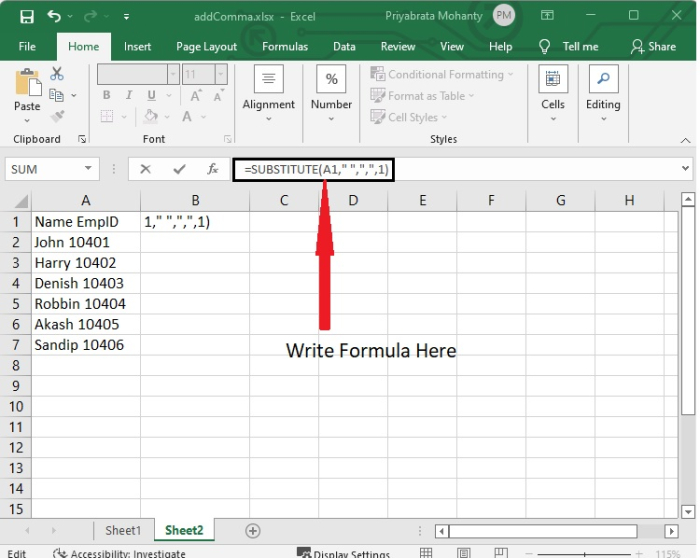### Step 2

When you press Enter, you will see that "Name, EmpID" is the result.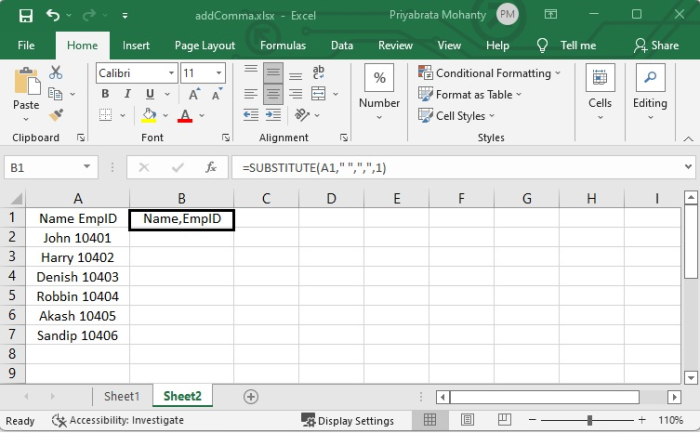You will obtain the desired outcome by selecting the cell in B1 and dragging it to the specified column below the B1 cell. This will cause the formula to be reflected in the other cells.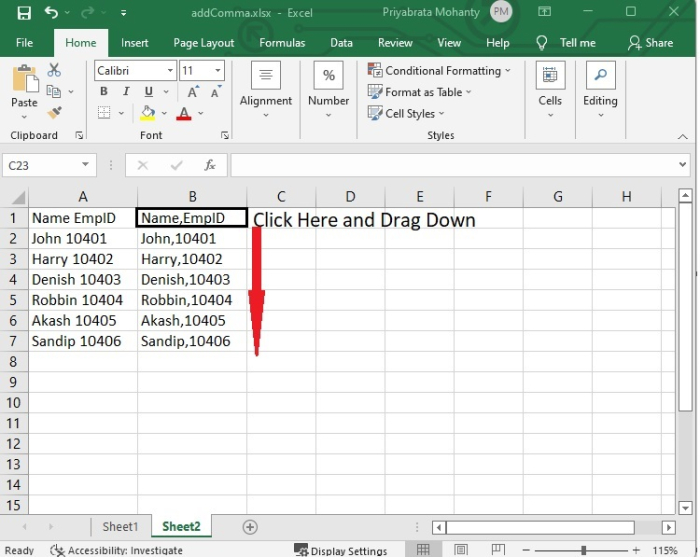## Conclusion

In this tutorial, you learned how to utilize the REPLACE function and the SUBSTITUTE function in Excel to insert a comma after the first word in a cell. You can use any of these two functions according to your requirement if and when required.